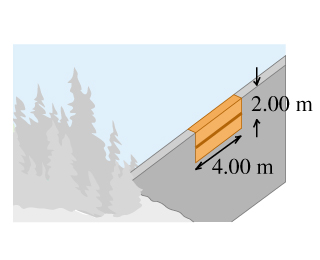# Problem: The upper edge of a gate in a dam runs along the water surface. The gate is 2.00 m high and 4.00 m wide and is hinged along a horizontal line through its center (the figure). Calculate the torque about the hinge arising from the force due to the water. (Hint: Calculate the torque on a thin, horizontal strip at a depth h and integrate this over the gate.)

###### Problem Details

The upper edge of a gate in a dam runs along the water surface. The gate is 2.00 m high and 4.00 m wide and is hinged along a horizontal line through its center (the figure). Calculate the torque about the hinge arising from the force due to the water. (Hint: Calculate the torque on a thin, horizontal strip at a depth h and integrate this over the gate.)Frequently Asked Questions

What scientific concept do you need to know in order to solve this problem?

Our tutors have indicated that to solve this problem you will need to apply the Calculating Force/Pressure via Integration concept. If you need more Calculating Force/Pressure via Integration practice, you can also practice Calculating Force/Pressure via Integration practice problems.

How long does this problem take to solve?

Our expert Physics tutor, Julia took 13 minutes and 56 seconds to solve this problem. You can follow their steps in the video explanation above.

What professor is this problem relevant for?

Based on our data, we think this problem is relevant for Professor Popov's class at UMICH.